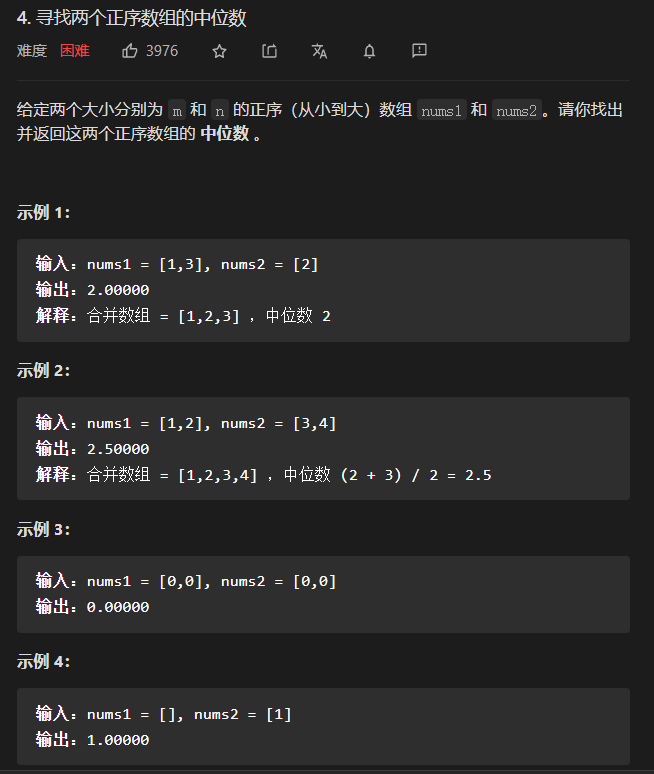# 寻找两个正序数组的中位数——题解class Solution {
public double findMedianSortedArrays(int[] nums1, int[] nums2) {
int leftLength = nums1.length;
int rightLength = nums2.length;
// 为了保证第一个数组比第二个数组小(或者相等)
if (leftLength > rightLength) {
return findMedianSortedArrays(nums2, nums1);
}
// 分割线左边的所有元素需要满足的个数 m + (n - m + 1) / 2;
// 两个数组长度之和为偶数时，当在长度之和上+1时，由于整除是向下取整，所以不会改变结果
// 两个数组长度之和为奇数时，按照分割线的左边比右边多一个元素的要求，此时在长度之和上+1，就会被2整除，会在原来的数
//的基础上+1，于是多出来的那个1就是左边比右边多出来的一个元素
int totalLeft = (leftLength + rightLength + 1) / 2;
// 在 nums1 的区间 [0, leftLength] 里查找恰当的分割线，
// 使得 nums1[i - 1] <= nums2[j] && nums2[j - 1] <= nums1[i]
int left = 0;
int right = leftLength;
// nums1[i - 1] <= nums2[j]
//  此处要求第一个数组中分割线的左边的值 不大于(小于等于) 第二个数组中分割线的右边的值
// nums2[j - 1] <= nums1[i]
//  此处要求第二个数组中分割线的左边的值 不大于(小于等于) 第一个数组中分割线的右边的值
// 循环条件结束的条件为指针重合，即分割线已找到
while (left < right) {
// 二分查找，此处为取第一个数组中左右指针下标的中位数，决定起始位置
// 此处+1首先是为了不出现死循环，即left永远小于right的情况
// left和right最小差距是1，此时下面的计算结果如果不加1会出现i一直=left的情况，而+1之后i才会=right
// 于是在left=i的时候可以破坏循环条件，其次下标+1还会保证下标不会越界，因为+1之后向上取整，保证了
// i不会取到0值，即i-1不会小于0
// 此时i也代表着在一个数组中左边的元素的个数
int i = left + (right - left + 1) / 2;
// 第一个数组中左边的元素个数确定后，用左边元素的总和-第一个数组中元素的总和=第二个元素中左边的元素的总和
// 此时j就是第二个元素中左边的元素的个数
int j = totalLeft - i;
// 此处用了nums1[i - 1] <= nums2[j]的取反，当第一个数组中分割线的左边的值大于第二个数组中分割线的右边的值
// 说明又指针应该左移，即-1
if (nums1[i - 1] > nums2[j]) {
// 下一轮搜索的区间 [left, i - 1]
right = i - 1;
// 此时说明条件满足，应当将左指针右移到i的位置，至于为什么是右移，请看i的定义
} else {
// 下一轮搜索的区间 [i, right]
left = i;
}
}
// 退出循环时left一定等于right，所以此时等于left和right都可以
// 为什么left一定不会大于right?因为left=i。
// 此时i代表分割线在第一个数组中所在的位置
// nums1[i]为第一个数组中分割线右边的第一个值
// nums[i-1]即第一个数组中分割线左边的第一个值
int i = left;
// 此时j代表分割线在第二个数组中的位置
// nums2[j]为第一个数组中分割线右边的第一个值
// nums2[j-1]即第一个数组中分割线左边的第一个值
int j = totalLeft - i;
// 当i=0时，说明第一个数组分割线左边没有值，为了不影响
// nums1[i - 1] <= nums2[j] 和 Math.max(nums1LeftMax, nums2LeftMax)
// 的判断，所以将它设置为int的最小值
int nums1LeftMax = i == 0 ? Integer.MIN_VALUE : nums1[i - 1];
// 等i=第一个数组的长度时，说明第一个数组分割线右边没有值，为了不影响
// nums2[j - 1] <= nums1[i] 和 Math.min(nums1RightMin, nums2RightMin)
// 的判断，所以将它设置为int的最大值
int nums1RightMin = i == leftLength ? Integer.MAX_VALUE : nums1[i];
// 当j=0时，说明第二个数组分割线左边没有值，为了不影响
// nums2[j - 1] <= nums1[i] 和 Math.max(nums1LeftMax, nums2LeftMax)
// 的判断，所以将它设置为int的最小值
int nums2LeftMax = j == 0 ? Integer.MIN_VALUE : nums2[j - 1];
// 等j=第二个数组的长度时，说明第二个数组分割线右边没有值，为了不影响
// nums1[i - 1] <= nums2[j] 和 Math.min(nums1RightMin, nums2RightMin)
// 的判断，所以将它设置为int的最大值
int nums2RightMin = j == rightLength ? Integer.MAX_VALUE : nums2[j];
// 如果两个数组的长度之和为奇数，直接返回两个数组在分割线左边的最大值即可
if (((leftLength + rightLength) % 2) == 1) {
return Math.max(nums1LeftMax, nums2LeftMax);
} else {
// 如果两个数组的长度之和为偶数，返回的是两个数组在左边的最大值和两个数组在右边的最小值的和的二分之一
// 此处不能被向下取整，所以要强制转换为double类型
return (double) ((Math.max(nums1LeftMax, nums2LeftMax) + Math.min(nums1RightMin, nums2RightMin))) / 2;
}
}
}posted @ 2021-04-15 22:00  崩天的勾玉  阅读(40)  评论(0编辑  收藏  举报